## Sample Problems from Chapter 11

Chapter 11: Compound Interest to e and i
The important thing about doing most math is that we really want more than the answer, we want to generalize the answer, to find the answer for any problem like we're workin on.
Ian, at age 11, came to me with the problem- what would his Dad pay in monthly installments on a house worth \$10,000 at a 10% annual interest, with a 30 year mortage- he really wanted a number for an answer. This got us working on the simpler problem of investing and finding the interest.

I must say that I don't know everything, and make a lot of mistakes, in spite of what my students might believe. So I wasn't able to give Ian a quick answer, but he and I worked very hard to solve his problem. We started this way.

1. Simple interest. Find the amount you have in the bank after 2 years if you put in \$1, at a 6% annual rate of interest. (It will always be an annual rate of interest with all the problems below.)
The Interest = Principal  *  rate  *  time
I(2 yr) = \$1 * .06 * 2 = \$.12 and the amount (A) you would have after 2 years
A = P + I = \$1 + \$.12 = \$1.12 Notice that the interest is not added each year to get a new principal.
2. Compound interest and 3, and 4. leading to a very important infinite sequence.
Here the interest is added after each compounding period.
Find the amount you have in the bank after 1 year, with a principal of \$1, at @6%, compounded semi-annually or 2 times per year.
The interest earned during the first (1/2) year = I(first 1/2 year) = \$1*.06*(1/2) = .06/2 (\$.03)
The Amount you have in the bank after the first (1/2) year
A = P + I =(1 + .06/2) = \$1.03 which will be the new principal.
I(2nd 1/2 year) = (1 + .06/2)* .06/2
A(after 2 (1/2)years)= P + I = (1 + .06/2) + (1 + .06/2)*.06/2
Factoring out the term (1 + .06/2), we get
A(after 2 (1/2)years) = (1 + .06/2)2 = \$1.124 (more than the simple interest after 2 years).
What would the amount you have in the bank after 1 year, putting in \$1, at 6%, compounded quarterly (4 times per year)?
What would the amount you have in the bank after 1 year, putting in \$1, at 6%, compounded monthly (12 times per year)?
What would the amount you have in the bank after 1 year, putting in \$1, at 6%, compounded daily (365 times per year)?
What would the amount you have in the bank after 1 year, putting in \$1, at 6%, compounded 10,000 times per year?
What would the amount you have in the bank after 1 year, putting in \$1, at 6%, compounded continuously, (an infinite number of times per year)?

New things, not in my books! See the answer page to find out what Kirsten and I did with graphing and exploring (1 + .07/x)x

5. Naming ex as an infinite series.
Using the binomial expansion from chapter 9, the first 5 terms of (A + B)n are: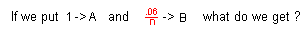6. Patterns with i
7. Leading to the most exciting single mathematical statement, that contains the 5 most important numbers in mathematics!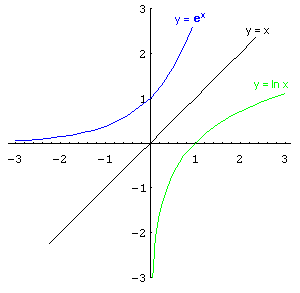This is not in my books, but happens regularly.When a student works on logs, as in Ch 13., I'll have them find ln(-1). Looking at the graph at the right, you can see that ln x is the inverse function of ex (they are mirror images of each other in the line y = x), but also there is no ln of a negative number! Now I'll have the student find ln(-1) in Derive and lo and behold we get ln x = i*Pi, which is not a real number but an imaginary number... and that shouldn't show up on our graph at the right. That means that ei*Pi = -1 by definition of logs. Adding 1 to each side we get

ei*Pi + 1 = 0.
Here are the 5 most important numbers in mathematics, in one statement!! When I first encountered this statement in college, I was so excited about it, that I did a watercolor painting of a circular rainbow with this statement in the center!
In my books I get this same statement using ex as an infinite series, then ei*x as an infinite series, eventually in terms of cos(x) and sin(x), then put Pi in for x.
8. Using iteration to do compound interest. Not done here.
9. One result of Ian playing with powers of powers.
Ian played with his calculator and powers of powers, during physics class. He came up with the following expression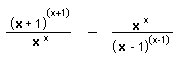. If x goes to infinity what does this go to? Click here to see how Ian figured this out.
10. Graphing powers of i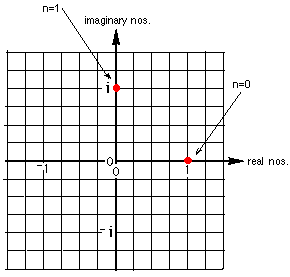Continue the graph. What do you notice?
Where is the point for i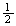?
11. Graphing powers of ( 1 + i )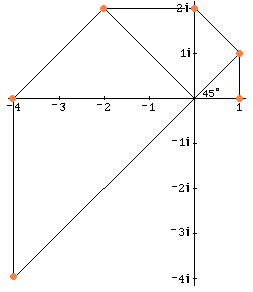Notice we get another spiral! (See chapter 6 for other spirals). If you double the angle, what happens to the length of the radius vector?
11a. Graph of iterating in, starting with n = i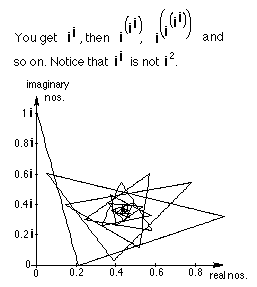Before Mathematica I never would have seen this graph! Notice, another spiral! (Actually there are 4 spirals here if you look at the endpoints of the segments). And ii is a real number! Amazing stuff here. One of the finest math sites on the internet, which has interactive applets, is IES in Japan. One applet inspired by the graph above is at I^I. You can drag the point at 0+1i
around the complex plane to get some amazing things!!

12. Back to Ian's problem.

Ways young people have solved these kinds of problems